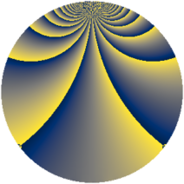# Properties

 Label 441.2.zLevel $441$ Weight $2$ Character orbit 441.z Rep. character $\chi_{441}(4,\cdot)$ Character field $\Q(\zeta_{21})$ Dimension $648$ Newform subspaces $1$ Sturm bound $112$ Trace bound $0$

# Related objects

## Defining parameters

 Level: $$N$$ $$=$$ $$441 = 3^{2} \cdot 7^{2}$$ Weight: $$k$$ $$=$$ $$2$$ Character orbit: $$[\chi]$$ $$=$$ 441.z (of order $$21$$ and degree $$12$$) Character conductor: $$\operatorname{cond}(\chi)$$ $$=$$ $$441$$ Character field: $$\Q(\zeta_{21})$$ Newform subspaces: $$1$$ Sturm bound: $$112$$ Trace bound: $$0$$

## Dimensions

The following table gives the dimensions of various subspaces of $$M_{2}(441, [\chi])$$.

Total New Old
Modular forms 696 696 0
Cusp forms 648 648 0
Eisenstein series 48 48 0

## Trace form

 $$648 q - 8 q^{2} - 13 q^{3} + 44 q^{4} + 3 q^{5} + 2 q^{6} - 7 q^{7} - 16 q^{8} - 39 q^{9} + O(q^{10})$$ $$648 q - 8 q^{2} - 13 q^{3} + 44 q^{4} + 3 q^{5} + 2 q^{6} - 7 q^{7} - 16 q^{8} - 39 q^{9} - 22 q^{10} - 5 q^{11} + 2 q^{12} - 4 q^{13} + 52 q^{14} - 4 q^{15} + 38 q^{16} - 37 q^{17} - 16 q^{18} - 14 q^{19} - 11 q^{20} - 6 q^{21} - q^{22} - 20 q^{23} - 26 q^{24} - 97 q^{25} - 44 q^{26} + 14 q^{27} - 22 q^{28} - 53 q^{29} - 9 q^{30} + 10 q^{31} - 10 q^{32} + 2 q^{33} - 7 q^{34} - 32 q^{35} - 22 q^{36} - 39 q^{37} + 59 q^{38} - 26 q^{39} - 19 q^{40} - 17 q^{41} - 83 q^{42} - q^{43} - 31 q^{44} + 81 q^{45} + 56 q^{46} + 50 q^{47} - 23 q^{48} - 19 q^{49} - 21 q^{50} - 30 q^{51} - 9 q^{52} + 50 q^{53} + 48 q^{54} + 20 q^{55} - 239 q^{56} + 33 q^{57} + 17 q^{58} - 37 q^{59} - 152 q^{60} - 105 q^{61} - 30 q^{62} + 6 q^{63} - 88 q^{64} - 5 q^{65} - 99 q^{66} + 13 q^{67} - 290 q^{68} + 64 q^{69} + 17 q^{70} - 19 q^{71} + 11 q^{72} - 40 q^{73} - 57 q^{74} + 69 q^{75} - 41 q^{76} + 19 q^{77} + 81 q^{78} + 13 q^{79} + 163 q^{80} - 179 q^{81} - 28 q^{82} + 73 q^{83} - 341 q^{84} - 10 q^{85} + 15 q^{86} + 13 q^{87} - 13 q^{88} - 104 q^{89} + 171 q^{90} - 49 q^{91} - 91 q^{92} - 275 q^{93} - 4 q^{94} - 29 q^{95} + 231 q^{96} - 11 q^{97} - 33 q^{98} + 62 q^{99} + O(q^{100})$$

## Decomposition of $$S_{2}^{\mathrm{new}}(441, [\chi])$$ into newform subspaces

Label Dim $A$ Field CM Traces $q$-expansion
$a_{2}$ $a_{3}$ $a_{5}$ $a_{7}$
441.2.z.a $648$ $3.521$ None $$-8$$ $$-13$$ $$3$$ $$-7$$